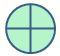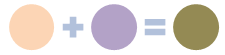## C# Math.Round Method

Math.Round method rounds a value to the nearest integer or to the specified number of fractional digits.

### How do you round a number to 2 decimal placesIn some situations you have to change the decimal places of a number limited to two digits. From the following section you can understand how to change the decimal places of decimal, float and double decimal places rounding to 2 digits.

## Round a float value to 2 decimal places

```float f = 10.123456F;
float fc = (float)Math.Round(f * 100f) / 100f;
MessageBox.Show(fc.ToString());
```

## Round a double value to 2 decimal places

```Double d = 100.123456;
Double dc = Math.Round((Double)d, 2);
MessageBox.Show(dc.ToString());
```

## Round a decimal value to 2 decimal places

```decimal d = 100.123456M;
decimal dc = Math.Round(d, 2);
MessageBox.Show(dc.ToString());
```

#### Output : 10.12The next few methods shows how to convert a number to a string and also restrict it to 2 decimal places.

```decimal d = 100.123456M;
MessageBox.Show(d.ToString("#.##"));
```

#### Output : 10.12

```decimal d = 100.123456M;
MessageBox.Show(d.ToString("F"));
```

#### Output : 10.12

```decimal d = 100.123456M;
MessageBox.Show(String.Format("{0:0.00}", d));
```

#### Output : 10.12NEXT.....Multiple Lines String